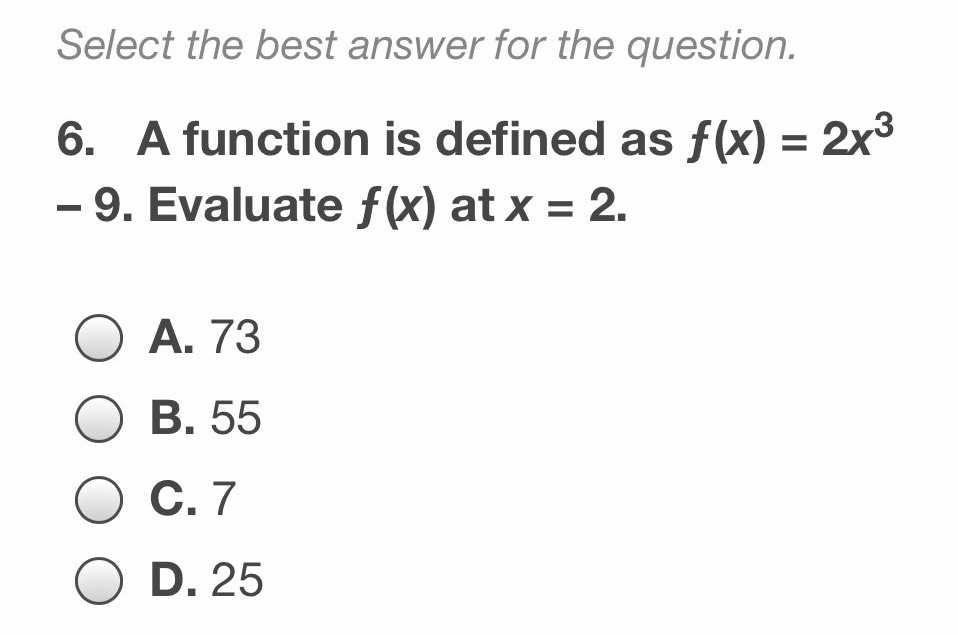### ¿Todavía tienes preguntas de matemáticas?

Pregunte a nuestros tutores expertos
Algebra
Pregunta6. A function is defined as $$f ( x ) = 2 x ^ { 3 }$$ - 9. Evaluate $$f ( x )$$ at $$x = 2$$ .

A. $$73$$

B. $$55$$

C. $$7$$

D. $$25$$